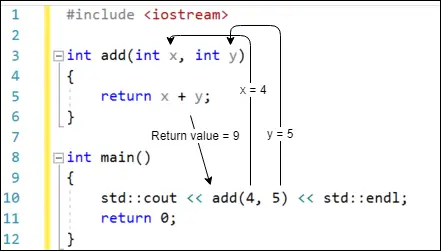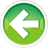# 2.3 — Introduction to function parameters and arguments

In the previous lesson, we learned that we could have a function return a value back to the function’s caller. We used that to create a modular getValueFromUser function that we used in this program:

However, what if we wanted to put the output line into its own function as well? You might try something like this:

This won’t compile, because function printDouble doesn’t know what identifier num is. You might try defining num as a variable inside function printDouble():

While this addresses the compiler error and makes the program compile-able, the program still doesn’t work correctly (it always prints “0 doubled is: 0”). The core of the problem here is that function printDouble doesn’t have a way to access the value the user entered.

We need some way to pass the value of variable num to function printDouble so that printDouble can use that value in the function body.

Function parameters and arguments

In many cases, it is useful to be able to pass information to a function being called, so that the function has data to work with. For example, if we wanted to write a function to add two numbers, we need some way to tell the function which two numbers to add when we call it. Otherwise, how would the function know what to add? We do that via function parameters and arguments.

A function parameter is a variable used in a function. Function parameters work almost identically to variables defined inside the function, but with one difference: they are always initialized with a value provided by the caller of the function.

Function parameters are defined in the function declaration by placing them in between the parenthesis after the function identifier, with multiple parameters being separated by commas.

Here’s some examples of functions with different numbers of parameters:

An argument is a value that is passed from the caller to the function when a function call is made:

Note that multiple arguments are also separated by commas.

How parameters and arguments work together

When a function is called, all of the parameters of the function are created as variables, and the value of each of the arguments is copied into the matching parameter. This process is called pass by value.

For example:

When function printValues is called with arguments 6 and 7, printValues‘s parameter x is created and assigned the value of 6, and printValues‘s parameter y is created and assigned the value of 7.

This results in the output:

```6
7
```

Note that the number of arguments must generally match the number of function parameters, or the compiler will throw an error. The argument passed to a function can be any valid expression (as the argument is essentially just an initializer for the parameter, and initializers can be any valid expression).

Fixing our challenge program

We now have the tool we need to fix the program we presented at the top of the lesson:

In this program, variable num is first initialized with the value entered by the user. Then, function printDouble is called, and the value of argument num is copied into the value parameter of function printDouble. Function printDouble then uses the value of parameter value.

Using return values as arguments

In the above problem, we can see that variable num is only used once, to transport the return value of function getValueFromUser to the argument of the call to function printDouble.

We can simplify the above example slightly as follows:

Now, we’re using the return value of function getValueFromUser directly as an argument to function printDouble!

Although this program is more concise (and makes it clear that the value read by the user will be used for nothing else), you may also find this “compact syntax” a bit hard to read. If you’re more comfortable sticking with the version that uses the variable instead, that’s fine.

A warning about function argument order of evaluation

The C++ specification does not define whether arguments are matched with parameters in left to right order or right to left order. When copying values, is of no consequence. However, if the arguments are function calls, then this can be problematic:

If the architectures evaluates left to right, a() will be called before b(). If the architecture evaluates right to left, b() will be called before a(). This may or not be of consequence, depending on what a() and b() do.

If it is important that one argument evaluate first, you can should explicitly define the order of execution, like so:

Warning

The C++ specification does not define whether function calls evaluate arguments left to right or right to left. Take care not to make function calls where argument order matters.

How parameters and return values work together

By using both parameters and a return value, we can create functions that take data as input, do some calculation with it, and return the value to the caller.

Here is an example of a very simple function that adds two numbers together and returns the result to the caller.

Execution starts at the top of main. When `add(4, 5)` is evaluated, function add is called, with parameter x being initialized with value 4, and parameter y being initialized with value 5.

The return statement in function add evaluates x + y to produce the value 9, which is then returned back to main. This value of 9 is then sent to std::cout to be printed on the console.

Output:

```9
```

In pictorial format:More examples

Let’s take a look at some more function calls:

This program produces the output:

```9
6
15
10
7
6
```

The first statement is straightforward.

In the second statement, the arguments are expressions that get evaluated before being passed. In this case, 1 + 2 evaluates to 3, so 3 is copied to parameter x. 3 * 4 evaluates to 12, so 12 is copied to parameter y. add(3, 12) resolves to 15.

The next pair of statements is relatively easy as well:

In this case, add() is called where the value of a is copied into both parameters x and y. Since a has value 5, add(a, a) = add(5, 5), which resolves to value 10.

Let’s take a look at the first tricky statement in the bunch:

When the function add is executed, the program needs to determine what the values for parameters x and y are. x is simple since we just passed it the integer 1. To get a value for parameter y, it needs to evaluate multiply(2, 3) first. The program calls multiply and initializes z = 2 and w = 3, so multiply(2, 3) returns the integer value 6. That return value of 6 can now be used to initial the y parameter of the add function. add(1, 6) returns the integer 7, which is then passed to std::cout for printing.

Put less verbosely:

The following statement looks tricky because one of the parameters given to add is another call to add.

But this case works exactly the same as the prior case. add(2, 3) resolves first, resulting in the return value of 5. Now it can resolve add(1, 5), which evaluates to the value 6, which is passed to std::cout for printing.

Less verbosely:

Conclusion

Function parameters and return values are the key mechanisms by which functions can be written in a reusable way, as it allows us to write functions that can perform tasks and return retrieved or calculated results back to the caller without knowing what the specific inputs or outputs are ahead of time.

Quiz time

Question #1

What’s wrong with this program fragment?

Show Solution

Question #2

What two things are wrong with this program fragment?

Show Solution

Question #3

What value does the following program print?

Show Solution

Question #4

Write a function called doubleNumber() that takes one integer parameter and returns twice the value passed in.

Show Solution

Question #5

5) Write a complete program that reads an integer from the user, doubles it using the doubleNumber() function you wrote for quiz answer 4, and then prints the doubled value out to the console.

Show Solution2.4 -- Introduction to local scopeIndex2.2 -- Function return values

### 1 comment to 2.3 — Introduction to function parameters and arguments

•nascardriver

dingdingding